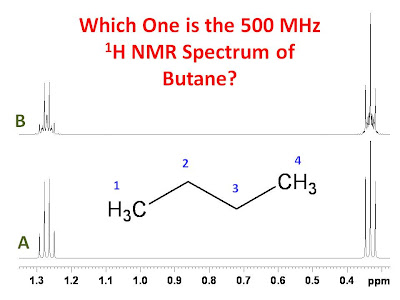## University of Ottawa NMR Facility Web Site

Please feel free to make suggestions for future posts by emailing Glenn Facey.

## Tuesday, August 4, 2009

### The 500 MHz 1H NMR Spectrum of Butane

n-Butane is a very simple molecule. Should it not then give a very simple 1H NMR spectrum? The figure below shows two calculated 500 MHz proton spectra for n-butane. Which spectrum most closely represents the true spectrum of n-butane?The answer is the very complicated spectrum B. The spectra were calculated with the following parameters:

Spectrum B
Spectrometer frequency = 500 MHz
δ1 = δ4 = 0.333 ppm
δ2 = δ3 = 1.271 ppm
3J12 = 3J34 = 7.11 Hz
4J13 = 4J24 = -0.07 Hz
3J23 = 6.77 Hz
LB = 0.5 Hz

Spectrum A
Spectrometer frequency = 500 MHz
δ1 = δ4 = 0.333 ppm
δ2 = δ3 = 1.271 ppm
3J12 = 3J34 = 7.11 Hz
4J13 = 4J24 = -0.07 Hz
3J23 = 0 Hz
LB = 0.5 Hz

The only difference between the simulations is that in spectrum B a coupling of 6.77 Hz was assumed between the two methylene groups whereas in spectrum A the same coupling was taken to be zero. The reason spectrum spectrum B is so complicated is that despite the fact that both the methyl groups and both the methylene groups are chemically equivalent, they are not magnetically equivalent. This is true for both spectrum A and spectrum B however, in spectrum A the second order effects are small based on the parameters used in the simulation.

Thank you to Adrian Dingle for inspiring me to create this post.

Sherwood Strategics said...

Thanks for peaking my interest! Your post came up in a tweet on my twitter account feed, and I followed the link to your page. Having done NMR spectroscopy, both solution and solid state, in grad school and as a post-doc, it's been about three years since I have used my training on a concept experiment. THanks for reviving some of my old learnings :) I appreciate it!

Gregory Darnell, M.S./Ph.D
Chicago, IL

Gaspard Huber said...

dear Glenn,